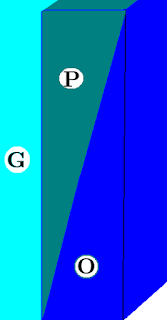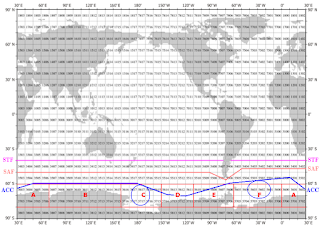## Thursday, November 15, 2018

### The Ghost Plumes - 3Fig. 1 'G'=glacier, 'P'=plume, 'O'=ocean
I. Background

In the previous post of this series I mentioned that the remaining work to be done on this project was to calculate the plume flow rate (The Ghost Plumes - 2).

I have finished a first pass at writing the software module that calculates potential plumes and quantities of melt water flow from them.

Here is the story of what is involved and how I did it.

II. The Area of Concern

Antarctica has the greatest quantity of ice on the planet.

That ice is "stored" in a vast ice sheet that covers the continent with ice streams which terminate quite often as tidewater glaciers when those ice streams very slowly but very surely meet the Southern Ocean.

There, they come in contact with seawater that is part of the largest current on planet Earth (follow the link at Fig. 2 for more information).Fig. 2 Current Events
The water flowing in that circumpolar current is warming, so it threatens a glacier population that holds back many meters of sea level rise (Antarctica 2.0 - 6; Appendices A, B, C, D, E, F).

III. The Ghost Plumes

While studying the theory about basal melt plumes, I noticed that the theorists (e.g.  O'Leary) did not deal with non-basal melt (thermodynamically spontaneous) plumes because they had their hands full dealing with basal melt plumes and how those plumes worked.

Thermodynamically spontaneous facial melt plumes were not mentioned, so facial melt was only mentioned in the basal melt sense (ibid).

Thermodynamically spontaneous facial melt plumes ("ghost plumes") showed up in calculations I was doing using the TEOS-10 function gsw_melting_ice_into_seawater.

I noticed that as I was processing billions of measurements, including those in WOD Zones along the Antarctic coastline, there were indications that the subsurface seawater was melting the tidewater glaciers at various and sundry depth levels.

The graphic at Fig. 1 depicts an idealized representation of the ghost plumes that I discovered (while not looking for them specifically).

These plumes spontaneously develop when heat spontaneously moves (2nd law of thermodynamics) from the seawater into the ice and causes the ice to melt into a plume.

IV. The Formulas

As I implied in the previous post ("Solving for the velocity of the plume flow is essential for determining the amount of melt water that is entering the ocean so as to contribute to sea level rise"), discovery of these ghost plumes was not the end of the matter.

I tried to redesign the formulas that deal with basal melt so they would work for ghost plumes.

It didn't happen because they were designed before TEOS-10 and therefore did not utilized the now official methods of properly calculating Conservative Temperature and Absolute Salinity.

They used salinity and temperature concepts that world oceanography bodies have escaped from, and provided free and open tools for such calculations (TEOS-10 Org).

Free from that past, I was able to use relatively simple formulas to do the necessary work.

I first noted that the difference between the density of the plume and the density of the ambient water was a source of energy, a force.

A force which we know as "buoyancy."

The formula for that force returns energy in "newtons" as follows:
buoyancy force (Newtons) = mass * g (force of gravity)
acceleration (m/s2) = buoyancy force / mass
cycle time (m/s2) = plume height / acceleration
cycle time (minutes) = cycle time (m/s2)) / 60
cycle time (hours) = cycle time (minutes) / 60
To make the calculations tight, I calculated the force of gravity (gsw_grav) and the mass at each depth level when the TEOS-10 functions indicated that the ice at that level was melting.

Next I calculated a flow coefficient and a drag coefficient.

The drag coefficient is a factor that arises when there is a depth level that did not completely melt according to the "gsw_melting_ice_into_seawater" function.

That layer would slow down the upward flow of deeper levels, so I subtracted the drag coefficent from the flow coefficient.

In today's appendices (Appendix A, B, C, D, E, F) you will come across some instances where the size of the plume in one year is the same or very close to another year, but the flow coefficient is different in a magnitude at odds with the magnitude one would expect.

That is because upon occasion there is less-than-complete melt in some layers sandwiched between the complete melt depth layers.

In those instances, the flow coefficient must be diminished by the drag coefficient.

If any readers would like the flow coefficient and drag coefficient logic ask in an email or comment.

If any readers see any problems with the logic please share and I will make corrections as needed.

V. The Appendices

Today's appendices are (Appendix A, B, C, D, E, and F).

In previous posts I supplied some appendices (see previous post appendices A, B, C, D, E, F) for only one zone because it would take an inordinate amount of blog space to include all of the zones involved.

To bolster today's post, I am including the summation values for all WOD Zones in all Antarctic Areas.

The data are summarized annually:
"Year: 2018: plume height (725 m), flow coefficient (0.0143624 m3 hr)
[10.4128 m3 hr * glacier width (m)]"
(example from Appendix F). But wait, there is more than meets the eye to this.

The  "10.4128 m3 hr" is the computation result for a one meter column of the plume, produced by multiplying the plume height by the flow coefficient.

One can derive the full flow of any glacier if one knows the glacier and the width of that glacier in a specific Antarctica area and/or WOD zone.

Just multiply the glacier width (m) by the one-meter-column value (e.g. 10.4128 * 100 for a 100 meter wide glacier with the same subsurface height).

VI. Outlet Glacier Width Potentials (Update)

I found a paper that gives maximum outlet glacier grounding line lengths for all the WOD Zones around Antarctica:
"Figure 4 shows the final grounded ice boundary produced by these procedures. It is 53 610 km long and contains 3 574 365 points at a 15-m resolution. The convoluted nature is less apparent at this scale, but for comparison’s sake, the length of the 72◦ latitude line is 12 350 km."

["53 610 km" x 0.13 (outlet gl %) = 6969.3 km (x 1000 = 6,969,300 m)
(6,969,300 m ÷ 36 (#zones per layer) = 193,591.6666 m max per zone)]
(Bindschadler et al 2011, PDF; The Cryosphere, 5, 569–588, 2011; at p. 575). The GLIMS database only gives 8 widths out of 509 outlet glaciers/ice streams around Antarctica.Bindschadler et al 2011 (Grounding line percentages)
So, I added the maximum spontaneous plume melt water capacity per hour for each zone that has in situ measurements for any year.

The potential addition to the melt water total is considerable.

The Appendices A, B, C, D, E, and F were updated accordingly.

VII. Conclusion

The most important thing to remember about utilizing this hypothesis is that it is a "telescope hypothesis."

By that I mean that this only presents a view from afar about potential dynamics in an area the size of a WOD zone (ten degrees latitude by ten degrees longitude).

And it is based on available measurements.

The bottom line is that those researchers who would want to validate or falsify the hypothesis could localize their search, could in effect make their search area smaller, and thereby do less work to find a target glacier.

The ghost plumes are "wiley wascals" as Wile E. Coyote discovered about the Road Runner, because the currents that are creating them are constantly changing as the oceans warm and oscillate.

This hypothesis is only set forth to get closer to the reality, to help fully solve all aspects of this issue.

And remember, this spontaneous plume flow is in addition to calving and basal melt plumes.

The next post in this series is here, the previous post in this series is here.

#### 1 comment:

1.Another aspect of the ghost plume phenomena is that the spontaneous thermodynamic melting can take place whether the tidewater portion of the glacier is advancing or retreating.